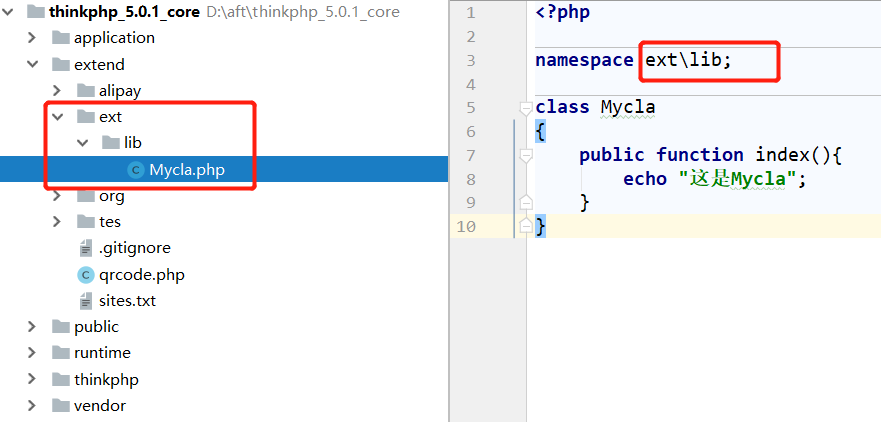# ThinkPHP5——引用vendor或extend里的第三方类（多种方法）

```namespace lib;

class Mycla
{
public function index(){
echo "这是Mycla";
}
}```

```\$myc = new \lib\Mycla();
\$myc->index();```

```use lib\Mycla;

\$myc = new Mycla();
\$myc->index();``````        // 引入 extend/qrcode.php；
// 助手函数
import('qrcode', EXTEND_PATH);
\$qrc = new \qrcode();
\$qrc->index();

// 引入 extend/tes/Ind.php；第三参数是后缀名，不加也可以
// 助手函数；第三个参数后缀名，不加也可以
import('tes.Ind', EXTEND_PATH);
\$ind = new \Ind();
\$ind->index();```

```// 引入 vendor/tes/Ind.php
// 助手函数
import('tes.Ind', VENDOR_PATH,'.php');
//助手函数，快速导入第三方框架类库
vendor('tes.Ind');

\$ind = new \Ind();
\$ind->index();```

```include_once '../extend/tes/Ind.php';
\$ind = new \Ind();
\$ind->index();```

`include_once '../../../extend/tes/Ind.php';`

extend/tes/conf.php；如果不写return，也可以直接调用\$student，最好预先定义不然报红(不定也不会报错)

```<?php
return \$student = [
"Id"    =>  1,
"name"  =>  "LHS",
"age"   =>  "21"
];```

```//预先定义，不然报红(注：不定义也不会报错)
//        \$student = [];
\$student = include_once '../extend/tes/conf.php';
print_r(\$student);    ```